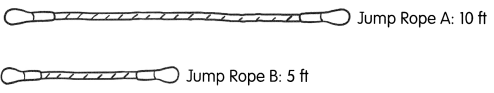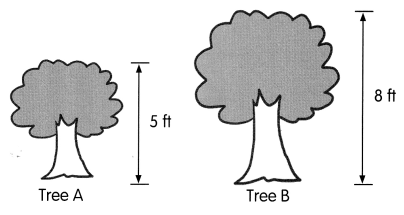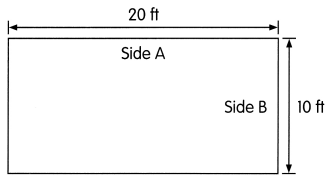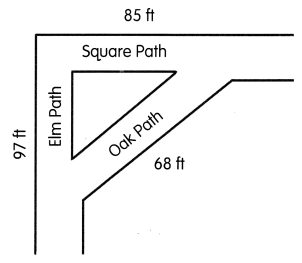# Math in Focus Grade 2 Chapter 13 Practice 2 Answer Key Comparing Lengths in Feet

This handy Math in Focus Grade 2 Workbook Answer Key Chapter 13 Practice 2 Comparing Lengths in Feet detailed solutions for the textbook questions.

## Math in Focus Grade 2 Chapter 13 Practice 2 Answer Key Comparing Lengths in Feet

Fill in the blanks.

Question 1.
Look at the two jump ropes.a. Which jump rope is longer? Jump Rope __________

Jump Rope A is longer than Jump Rope B

b. How much longer is it? __________ ft

Jump Rope A is 5 ft longer than Jump Rope B.

Question 2.
Look at the trees.a. Which tree is taller? Tree ___________

Tree B is taller than Tree A.

b. How much taller is it? ___________ ft

Tree B is 3 ft taller than Tree A.

Answer the questions. Look at the sides of the rectangle.Question 3.
Which is longer, Side A or Side B? Side ___________

Side A is longer than Side B.

Question 4.
How much longer is it? ___________ ft

Side A is 10 ft longer than Side B.

Question 5.
Look at the paths.a. Which path is the longest?

Elm path is longer than Oak path and Square path.

b. How much longer is Elm Path than Square Path? _________ ft